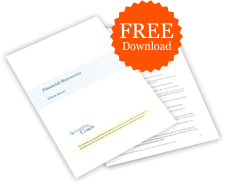# What would cause a decrease in accumulated depreciation?

## Definition of Accumulated Depreciation

Accumulated depreciation is the cumulative amount of depreciation taken since a depreciable asset was put into service. The balance sheet asset account Accumulated Depreciation is a contra asset account since it has a credit balance. Whenever depreciation expense is recorded (with a debit entry), Accumulated Depreciation is credited.

The combination (or net) of the asset's debit balance and the asset's accumulated depreciation is referred to as the asset's book value or carrying value.

When the depreciable asset is sold, scrapped, or retired, its accumulated depreciation and its cost are removed from the respective accounts. (Any difference between the book value and the amount received is recorded as a gain or loss on the disposal.)

## Example of a Decrease in Accumulated Depreciation

Assume that a company purchased new equipment 5 years ago at a cost of \$210,000. The asset had a useful life of 7 years and no salvage value. As a result, each year the company debited Deprecation Expense for \$30,000 and credited Accumulated Depreciation for \$30,000. Therefore, after 5 years, the Equipment account has the cost of \$210,000 and the Accumulated Depreciation account has a credit balance of \$150,000. (Therefore, the asset's book value or carrying value is \$60,000.)

On the next day, the company sells the equipment for \$32,000. The necessary entry will:

• Remove the equipment's cost from the Equipment account with a credit of \$210,000
• Remove the equipment's accumulated depreciation from the Accumulated Depreciation account with a debit of \$150,000
• Record the receipt of \$32,000 with a debit of \$32,000 to the Cash account
• Record the Loss on Sale of Equipment with a debit of \$28,000 since the company removed the equipment's carrying value of \$60,000 and received only \$32,000 of cash

Some accounting textbooks state that the cost of an expenditure that extends the useful life of an asset should be debited to the accumulated depreciation account instead of the asset account. Such an entry will also reduce the credit balance in the accumulated depreciation account.### Free Financial Statements Cheat Sheet

546,033
Subscribers
You are already subscribed. This offer is not available to existing subscribers.
Error: You have unsubscribed from this list.